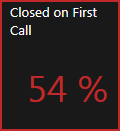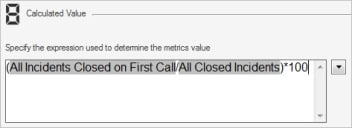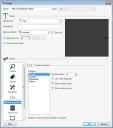# Metric Example

First Call Resolution (FCR) rate is a Metric that measures the percentage of Incidents that are closed on the first call.

FCR rate could be calculated like this:

AllIncidentsClosedonFirstCall/AllClosedIncidents

## Metric Definition

The Metric definition would look like this:

All IncidentsClosed on FirstCall/AllClosed Incidents

and would generate a decimal value like this:

.54

Once created, you could use this Metric on a Gauge Widget, Expression, or Query.

## Use the Metric in a Gauge Widget

Here is what the Metric could look like in a Gauge Widget:Note that on the Gauge, the Metric is formatted as a percentage.

You could also build the percentage into the Metric (example: (AllIncidentsClosedonFirstCall/AllClosedIncidents) *100); however, you would then need to format the value as a number; otherwise, you'll get an unexpected result (percentage of percentage).## Use the Metric in an Expression

You could then use the same Metric in an Expression to conditionally change the border color of the Gauge Widget to an Alert when the percentage falls below a satisfactory level (example: 75 percent).